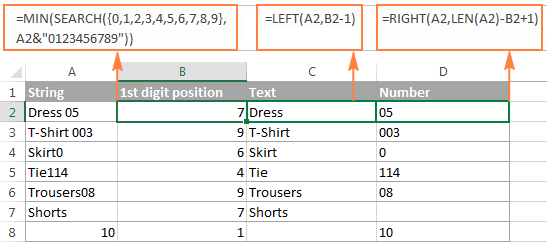# How To Split Text String At Specific Character In Excel

Oct 17, 2020 • edited Oct 20, 2020Below we will look at a program in Excel VBA that separates strings.

Situation:Place a command button on your worksheet and add the following code lines:

1. First, we declare a variable called fullname of type String, a variable called commaposition of type Integer, and a variable called i of type Integer.

Dim fullname As String, commaposition As Integer, i As Integer

The problem we are dealing with is that we need to tell Excel VBA where we want to separate the string. In case of Smith, Mike the comma is at position 6 while in case of Williams, Janet the comma is at position 9.

2. We use a loop to execute the operations on each name entered in Excel. First, we initialize the variable fullname. Next, we use the Instr function to find the position of the comma.

For i = 2 To 7

fullname = Cells(i, 1).Value

commaposition = InStr(fullname, ",")

3. Finally, we want to write the part after the comma to column B and the part in front of the comma to column C. You can achieve this by adding the lines:

Cells(i, 2).Value = Mid(fullname, commaposition + 2)

Cells(i, 3).Value = Left(fullname, commaposition - 1)

Mid(fullname, commaposition + 2) means we want the part of fullname starting at character ‘commaposition + 2’ (this is exactly the first name).

Left(fullname, commaposition - 1) means we want the part of fullname starting at the beginning until character ‘commaposition- 1’ (this is exactly the last name).

4. Don't forget to close the loop.

Next i

5. Add six names separated by a comma and space to Range("A2:A7").

6. Test the program.

Result:#Tutorial#How To#VBA#String Manipulation

How To Sort By Number In Excel

How To Use Excel VBA Select Case